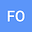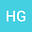A calculator proposal for estimating refractive error after 3 years using the onset biometric values in primary school children: a cohort study
••Haluk Huseyin Gürsoy
Eskisehir Osmangazi University
Author Profile## Abstract

Aim To evaluate consecutive measurements of the biometric parameters, age, and refraction error in a Turkish population at primary school age. Materials and Methods A total of 197 children aged between 7-12 years were included. The data of three consecutive measurements of children, who were examined at least once a year for three years using both cycloplegic auto-refractometry and optical biometry, were used in this retrospective study. Spherical equivalent <-0.50D was considered to be myopic; >+0.75D was considered to be hypermetropic. Age, gender, body mass index, spherical equivalent, axial length, anterior chamber depth, central corneal thickness, keratometry, and lens thickness were analyzed. The onset data obtained in 2013 whereas, the final data were from 2015. Logistic and Cox regression analyses were performed (p<0.05). Results The mean of the onset and the final spherical equivalents were 0.19D (0.56), and 0.08D (0.80), respectively. The myopia prevalence was increased among refractive errors in observation periods (univariable analysis p=0.029; multivariable analysis p=0.017). The onset axial length (HR:4.55, 95%CI:2.87-7.24, p<0.001), keratometry (HR:2.04, 95%CI:1.55-2.67, p<0.001) and age (HR:0.73, 95%CI: 0.57-0.92, p=0.009) correlated myopia progression. To calculate the estimated spherical equivalent, the onset data were included in the logistic regression model. The onset data of spherical equivalent (β=0.916, p<0.001), axial length (β=-0.451, p<0.001), anterior chamber depth (β=0.430, p=0.005) and keratometry (β=-0.172, p<0.001) were found to be significantly associated with the mean SE at the final data. Conclusions To calculate the estimated spherical equivalent following three years, an equation was proposed. The estimated refractive error of children can be calculated by using the proposed equation with the associated onset optical parameters.
26 Apr 2021Submitted to International Journal of Clinical Practice
27 Apr 2021Assigned to Editor
27 Apr 2021Submission Checks Completed
29 Apr 2021Reviewer(s) Assigned
18 May 2021Review(s) Completed, Editorial Evaluation Pending
27 Oct 2021Editorial Decision: Revise Minor